#### Chapter 24 Measure of Central Tendency R.D. Sharma Solutions for Class 9th Exercise 24.2

Exercise 24.2

1.
Calculate the mean for the following distribution:
 x: 5 6 7 8 9 f: 4 8 14 11 3

Solution

The given distribution in tabulated form is
 x: 5 6 7 8 9 f: 4 8 14 11 3
Prepare the following frequency table of which the first column consists of the values of the variate and the second column the corresponding frequencies. Multiply the frequency of each row with the corresponding values of variable to obtain the third column containing fixi.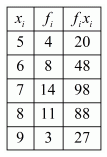Therefore,
Find the sum of all entries in the second and third column to obtain N and ∑fixi respectively.2. Find the mean of the following data:
 x: 19 21 23 25 27 29 31 f: 13 15 16 18 16 15 13

Solution

The given distribution in tabulated form is
 x: 19 21 23 25 27 29 31 f: 13 15 16 18 16 15 13

Prepare the following frequency table of which the first column consists of the values of the variate and the second column the corresponding frequencies. Multiply the frequency of each row with the corresponding values of variable to obtain the third column containing fixi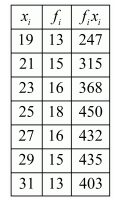Find the sum of all entries in the second and third column to obtain N and ∑fixi respectively. Therefore,3. The mean of the following data is 20.6. Find the value of p
 x: 10 15 p 25 35 f: 3 10 25 7 5

Solution

The given distribution in tabulated form is
 x: 10 15 p 25 35 f: 3 10 25 7 5

We have to find the value of p using the information that the mean of the distribution is 20.6.
Prepare the following frequency table of which the first column consists of the values of the variate and the second column the corresponding frequencies. Multiply the frequency of each row with the
corresponding values of variable to obtain the third column containing fixi.Find the sum of all entries in the second and third column to obtain N and ∑fixi respectively. Therefore,4. If the mean of the following data is 15, find p
 x: 5 10 15 20 25 f: 6 p 6 10 5

Solution

The given data in tabulated form is
 x: 5 10 15 20 25 f: 6 p 6 10 5

We have to find the value of p using the information that the mean of the data is 15. Prepare the following frequency table of which the first column consists of the values of the variate and the second column the corresponding frequencies. Multiply the frequency of each row with the corresponding values of variable to obtain the third column containing fixi.Find the sum of all entries in the second and third column to obtain N and ∑fixi respectively. Therefore,5. Find the value of p for the following distribution whose mean is 16.6.

 x: 8 12 15 p 20 25 30 f: 12 16 20 24 16 8 4

Solution

The given distribution in tabulated form is
 x: 8 12 15 p 20 25 30 f: 12 16 20 24 16 8 4
We have to find the value of p using the information that the mean of the distribution is 16.6. Prepare the following frequency table of which the first column consists of the values of the variate and the second column the corresponding frequencies. Multiply the frequency of each row with the corresponding values of variable to obtain the third column containing fixi .Find the sum of all entries in the second and third column to obtain N and ∑fixi respectively. Therefore,6. Find the missing value of p for the following distribution whose mean is 12.58.
 x: 5 8 10 12 p 20 25 f: 2 5 8 22 7 4 2

Solution

The given distribution in tabulated form is
 x: 5 8 10 12 p 20 25 f: 2 5 8 22 7 4 2

We have to find the value of p using the information that the mean of the distribution is 12.58.
Prepare the following frequency table of which the first column consists of the values of the variate and the second column the corresponding frequencies. Multiply the frequency of each row with the corresponding values of variable to obtain the third column containing fixi.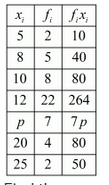Find the sum of all entries in the second and third column to obtain and ∑fixi respectively. Therefore,7. Find the missing frequency (p) for the following distribution whose mean is 7.68
 x: 3 5 7 9 11 13 f: 6 8 15 p 8 4

Solution

The given distribution in tabulated form is
 x: 3 5 7 9 11 13 f: 6 8 15 p 8 4

We have to find the value of p using the information that the mean of the distribution is 7.68. Prepare the following frequency table of which the first column consists of the values of the variate and the second column the corresponding frequencies. Multiply the frequency of each row with the corresponding values of variable to obtain the third column containing fixi.Find the sum of all entries in the second and third column to obtain N and fixi respectively. Therefore,8. Find the value of p, if the mean of the following distribution is 20.
 x: 15 17 19 20+p 23 f: 2 3 4 5p 6

Solution

The given data in tabulated form is
 x: 15 17 19 20+p 23 f: 2 3 4 5p 6

We have to find the value of p using the information that the mean of the data is 20. Prepare the following frequency table of which the first column consists of the values of the variate and the second column the corresponding frequencies. Multiply the frequency of each row with the corresponding values of variable to obtain the third column containing  fixi .Find the sum of all entries in the second and third column to obtain N and ∑fixi respectively. Therefore,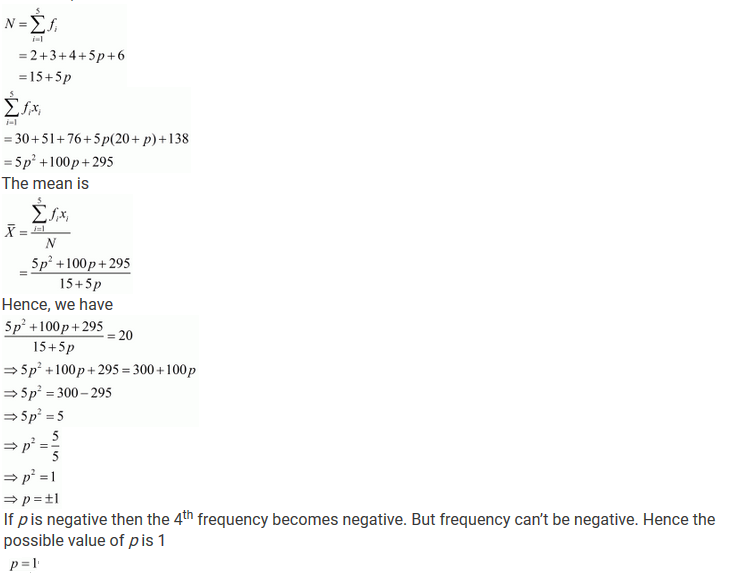9. Find the mean of the following distribution:
 x: 10 12 20 25 35 f: 3 10 15 7 5

Solution

The given distribution in tabulated form is
 x: 10 12 20 25 35 f: 3 10 15 7 5

Prepare the following frequency table of which the first column consists of the values of the variate and the second column the corresponding frequencies. Multiply the frequency of each row with the
corresponding values of variable to obtain the third column containing fixi. Find the sum of all entries in the second and third column to obtain N and ∑fixi respectively.Find the sum of all entries in the second and third column to obtain N and ∑fixi respectively. Therefore,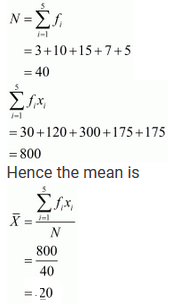10. Candidates of four schools appear in a mathematics test. The data were as follows: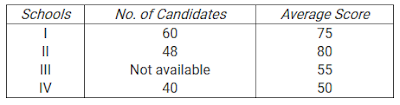If the average score of the candidates of all the four schools is 66, find the number of candidates that appeared from school III.

Solution

Let the number of candidates appeared from school III is p.
Then the given data can be tabulated asThe row of x denotes the average scores and the row of f denotes the number of candidates. We have to find the value of p using the information that the average score of all the four schools is 66. Prepare the following frequency table of which the first column consists of the average scores and the second column the number of candidate (frequencies). Multiply the frequency of each row with the corresponding average scores to obtain the third column containing fixi.Find the sum of all entries in the second and third column to obtain N and ∑fixi. respectively. Therefore,11. Five coins were simultaneously tossed 1000 times and at each toss the number of heads were observed. The number of tosses during which 0, 1, 2, 3,4 and 5 heads were obtained are shown in the table below. Find the mean number of heads per toss.Solution

The given data can be tabulated in the formThe row of x denotes the number of heads per toss and the row of f denotes the number of tosses.
Prepare the following frequency table of which the first column consists of the number of heads and the second column the number of tosses (frequencies). Multiply the frequency of each row with the corresponding number of heads to obtain the third column containing fixi.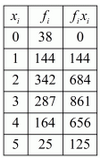Find the sum of all entries in the second and third column to obtain N (already given in the question) and fixi. respectively. Therefore,12. Find the missing frequencies in the following frequency distribution if it is known that the mean of the distribution is 50.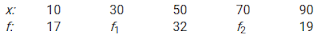Solution

The given distribution in tabulated form is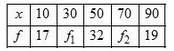We have to find the value of missing frequencies f1 and f2, using the information that the mean of the distribution is 50 and the total frequency is 120. Prepare the following frequency table of which the first column consists of the values of the variate and the second column the corresponding frequencies. Multiply the frequency of each row with the corresponding values of variable to obtain the third column containing fixi .Find the sum of all entries in the second and third column to obtain N and ∑fixi. respectively. Therefore,